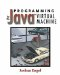# 10.1 Expressions and Statements

An expression is a fragment of Java code that yields a value. Expressions include field references, method calls, arithmetic operations, and constants. Expressions may be built up from other expressions. Some examples of expressions are listed in Table 10.1.

A statement is a unit of code that does not yield a value. Instead, it affects program state by producing some sort of side effect. Statements can set field values, control the flow of execution, or terminate methods, among other things. Statements usually end with semicolons (;). Some kinds of statements are composed of expressions or other statements. Sometimes a statement is composed of nothing but an expression. For example,

##### Table 10.1. Examples of Java expressions
Expression Explanation
"A String" Constant expression of type String
2+2 Arithmetic expression composed of two constants combined with the + operator
x*x Arithmetic expression composed of two field-reference subexpressions combined with the * operator
x*x+y*y Expression composed of two subexpressions, x*x and y*y, combined with the + operator
Math.sqrt(x*x+y*y) Method-call expression with one subexpression, x*x+y*y
(String) cache.get(key) Cast expression made from a method-call expression. The method-call expression has two subexpressions, cache and key, which are combined into a single method-call expression.

` System.out.println("Hello, world"); `

This statement consists of nothing but a method-call expression followed by a semicolon. The method-call expression has two subexpressions: System.out (a field-reference expression) and "Hello, world" (a constant String expression). The value of the expression is void, which means that it doesn't really yield a value when evaluated.

Following is another example of a statement:

` i = i+1; `

This statement is made up of the expression i = i+1. The value of that expression is the new value of i. By adding a semicolon (;) at the end, it becomes a statement.

You may put several statements together using braces ({}) to make a block, or compound statement. A compound statement doesn't have a semicolon at the end of it. The effect of a compound statement is the cumulative effect of all the statements within it executed sequentially. For example, this is a block with three statements:

` {    System.out.print(i + " ");    int fact = factorial(i);    System.out.println(fact); } `

Some kinds of statements, such as if, for, and while, include a substatement:

` // Print "Hello, world" 10 times for(int i = 0; i < 10; i++)    System.out.println("Hello, world"); `

A for statement may use a compound statement as its substatement:

` for(int i = 0; i < 10; i++) {                          // Braces denote a compound statement    System.out.println(i);    int i = factorial(i);    System.out.println(fact); } `Programming for the Javaв„ў Virtual Machine
ISBN: 0201309726
EAN: 2147483647
Year: 1998
Pages: 158
Authors: Joshua Engel

Similar book on Amazon

flylib.com © 2008-2017.
If you may any questions please contact us: flylib@qtcs.net